# Localizing visual gamma and cortico-muscular coherence using DICS

## Introduction

In this tutorial we will continue working on the combined visual and motor task dataset (Schoffelen, Poort, Oostenveld, & Fries (2011) Selective Movement Preparation Is Subserved by Selective Increases in Corticomuscular Gamma-Band Coherence. J Neurosci. 31(18):6750-6758) described in the channel-level analysis tutorial.

In this tutorial you will learn about applying beamformer techniques in the frequency domain. You will learn how to compute an appropriate head model and lead field matrix, how to compute appropriate time-frequency windows, and how to contrast the effect of interest against some control/baseline. Also, you will play around with several options for plotting the results overlaid on a structural MRI. Finally, you will apply the results from the sensor-level coherence analysis and localize sources that are coherent with the EMG signals.

It is expected that you understand the previous steps of preprocessing and filtering and understand the essence of (time-)frequency analysis of the sensor data. Some understanding of the options for computing the head model and forward lead field is also useful. Also you should be at least familiar with how to compute coherence on the sensor-level data before diving into the source-level.

This tutorial will not cover the time-domain option for LCMV/SAM beamformers nor beamformers applied to evoked/averaged data (although see the appendix and here for an example). Also, we will not provide a full-fledged background on coherence or other connectivity measures. For more information on coherence, you should either read the sensor level tutorial or the coherence tutorial. Other connectivity measures such as Granger causality and a more elaborate explanation of undirected and directed connectivity analysis can be found in the connectivity tutorial.

## Background

In the sensor-level tutorial we found gamma-band oscillations over occipital MEG channels during visual stimulation. Furthermore, we identified cortico-muscular coherence between the EMG and MEG channels over the contralateral MEG channels. The goal of this tutorial is to localize sources responsible for this oscillatory activity. We will use a beamformer, which is a adaptive spatial filter. Scanning with the beamformer over the whole brain allows us to estimate the activity everywhere in the brain. The filter is based on minimizing the source power (or variance) at a given location, subject to ‘unit-gain constraint’. This latter part means that, if a hypothetical source that projects to the sensors had a power of amplitude 1, the inverse filter applied to the sensor level representation would then reconstruct the source power with strength 1 at the location of the hypothetical source. Beamforming assumes that sources in different parts of the brain are not strongly temporally correlated.

The brain is divided in a regular three dimensional grid and the source strength for each grid point is computed. The method applied in this example is termed Dynamical Imaging of Coherent Sources (DICS) and the estimates are calculated in the frequency domain (Gross et al. 2001). Other beamformer methods rely on source estimates calculated in the time domain, e.g., the Linearly Constrained Minimum Variance (LCMV) and Synthetic Aperture Magnetometry (SAM) methods (van Veen et al., 1997; Robinson and Cheyne, 1997). These methods produce a 3D spatial distribution of the power of the neuronal sources. This distribution is then overlaid on a structural image of the subject’s brain. These distributions of source power can then be subjected to statistical analysis. It is always ideal to contrast the activity of interest against some control/baseline activity. Options for this will be discussed below, but it is best to keep this in mind when designing your experiment from the start, rather than struggle to find a suitable control/baseline after data collection.

When conducting a multiple-subject study, it is essential that averaging over subjects does not violate any statistical assumption. One of these assumptions is that subject’s sources are represented in a common space, i.e. an averaged grid point represents the estimate of the same brain region across subjects. One way to get subjects in a common space is by spatially deforming and interpolating the source reconstruction after beamforming. However, we will use (and recommend) an alternative way that does not require interpolation. Prior to source estimation we construct a regular grid in MNI template space and spatially deform this grid to each of the individual subjects (note that you will only have the data from one subject here). The beamformer estimation is done on the direct grid mapped to MNI space, so that the results can be compared over subjects. This procedure is explained in detail in this example code. Creating the MNI template grid only needs to be done once, and the result is provided in the fieldtrip/template directory. We strongly suggest that you have a quick (but thorough) look at the example code page and understand the essence of what is being done there anyway!

The tutorial is split into three parts. In the first part of the tutorial, we will explain how to compute the forward and inverse model, which is the fundamental basic for source level analysis. In the second part, we will localize the sources responsible for the posterior gamma activity upon visual stimulation. In the third part of the tutorial, we will compute coherence to study the oscillatory synchrony between two sources in the brain. This is computed in the frequency domain by normalizing the magnitude of the summed cross-spectral density between two signals by their respective power. For each frequency bin the coherence value is a number between 0 and 1. The coherence values reflect the consistency of the phase difference between the two signals at a given frequency. In the dataset we will analyze the subject was required to maintain an isometric contraction of a forearm muscle. The example in this session covers thus cortico-muscular coherence on source level. The same principles, however, apply to cortico-cortical coherence, for which the interested reader can have a look at another tutorial.

## Procedure

In the first part of this tutorial we will use the anatomical data to prepare the source analysis. This involve

Next, we head out to investigate the response to the visual stimulation. We will localize the sources of the visual gamma-band activity following the following step

In the third part we shift our attention to the motor task in this dataset. We will compute the spatial distribution of the cortico-muscular coherence over the whole brain using a very similar analysis pipeline.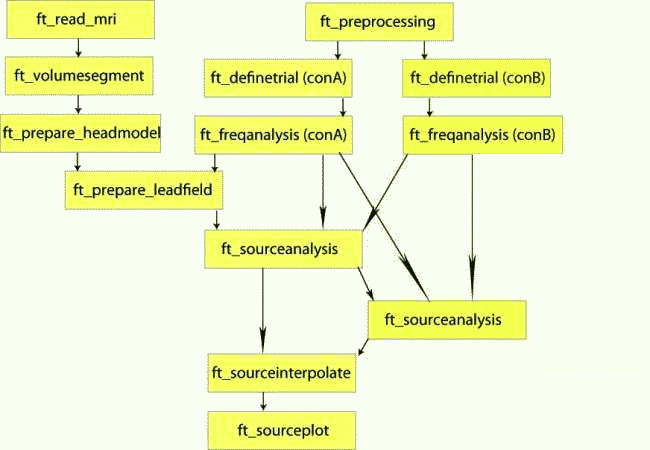## Preparing the data and the forward and inverse model

``````load subjectK
``````

Since we are not interested in the difference between left and right hand responses at this moment, we combine data from the left-hand and the right-hand response conditio

``````data_combined = ft_appenddata([], data_left, data_right);
``````

That is it with the data for now. We will now turn to preparing the head- and sourcemodel.

The first requirement for the source reconstruction procedure is that we need a forward model. The forward model allows us to calculate the distribution of the magnetic field on the MEG sensors given a hypothetical current distribution. The forward models for MEG are typically constructed for each subject individually, taking the position and especially the size of the head into account. The size of the head determines the distance between brain and MEG sensors, the larger the distance, the weaker the cortical sources will be observed by the MEG sensors. If you were to use a single head model for all subjects, the variance in signal strength that is due to the differences in distance would not be explained by the model, causing unexplained variance over subjects and reduced statistical sensitivity.

There are many types of forward models that, to various degrees, take the individual anatomy into account. We will here use a semi-realistic head model developed by Nolte (2003). It is based on a correction of the lead field for a spherical volume conductor by a superposition of basis functions, gradients of harmonic functions constructed from spherical harmonics.

The first step in constructing the forward model is to find the brain surface from the subjects MRI. You can download the MRI subjectK.mri from our download server. Each of the voxels of the anatomical MRI is assigned to a tissue class, this procedure is termed segmentation. Note that making the segmentation is quite time consuming.

``````load segmentedmri
``````

Otherwise, the segmentation involves ft_volumesegment which makes use of SPM. Note that you don’t need a separate SPM installation, the required SPM-files are included in the FieldTrip release in the fieldtripXXX/external/spm12 directory)):

``````mri = ft_read_mri('subjectK.mri');

cfg          = [];
segmentedmri = ft_volumesegment(cfg, mri);
``````

Note that the anatomical MRI has already been aligned to the coordinate system of the CTF MEG system, according to the anatomical landmarks (nasion, left and right ear canal) using ft_volumerealign. The location of these anatomical fiducials relative to the head were determined both in the MEG measurement (with localizer coils) and in the MRI scan (with vitamin E capsules). Having the fiducials in both measurements allows the data to be aligned to each other.

You can check whether the segmentation was successful by callin

``````% add anatomical information to the segmentation
segmentedmri.transform = mri.transform;
segmentedmri.anatomy   = mri.anatomy;

cfg              = [];
cfg.funparameter = 'gray';
ft_sourceplot(cfg, segmentedmri);
``````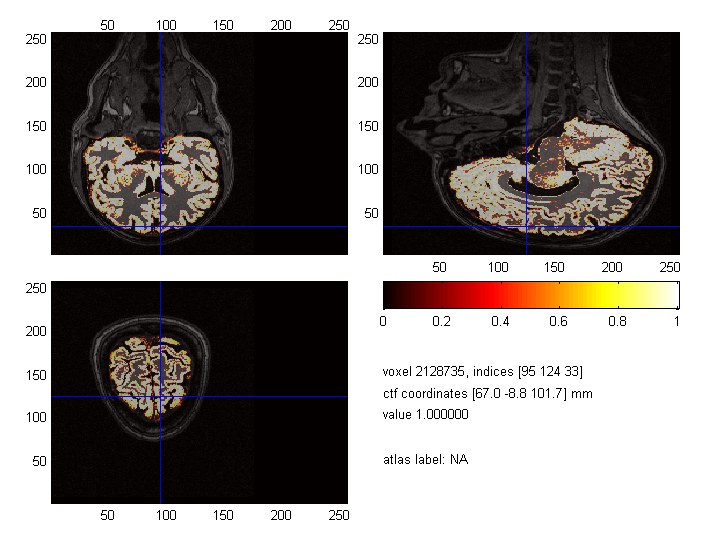Figure: The segmented MRI and the original MRI on top of each other. If everything went well, there is a perfect overlap between these two!

If the yellow-greyish brain shows up in the subject’s heads, everything went fine as shown in above Figure. Otherwise, it might be that you need to flip either of the three dimensions or that some unit conversion went wrong before segmenting the MRI.

You might wonder why the anatomical MRI shows upside down: this is a frequently asked question.

Now prepare the head model from the segmented brain surface

``````cfg        = [];
cfg.method = 'singleshell';
``````

Note that the head model can also be referred to as the volume conduction model, those two things refer to the same. Since we plan to use the head model on various locations throughout this tutorial, it might be wise to save it for now:

``````save hdm hdm
``````

If you want to do a source reconstruction of EEG data, you have to pay special attention to the referencing. The forward model will be computed with a common average reference (except in some rare cases like with bipolar iEEG electrode montages), i.e. the mean value of the forward model over all electrodes is zero. Consequently, this also has to hold for your data.

Prior to doing the spectral decomposition with ft_freqanalysis you have to ensure with ft_preprocessing that all channels are re-referenced to the common average reference.

Furthermore, after selecting the channels you want to use in the source reconstruction (excluding bad and absent channels) and after re-referencing them, you should not make sub-selections of channels any more and discard channels, as that would cause the data not be average referenced any more.

Why might a single sphere model be inadequate for performing beamformer estimates?

### Computing the sourcemodel

Following the construction of the volume conduction model, we need to discretize the brain into a source model or grid. For each grid point in the brain, the lead field matrix is calculated later. When constructing the source model, you might want to keep in mind that averaging and statistics over subjects can only be done if the individual subjects source reconstructed results are mapped onto a common space. Therefore, we will use a regular (isotropic) grid in MNI space and spatially deform this grid to the individual subjects brain. The following code loads the template grid that is included in the FieldTrip release:

``````% this returns the location where FieldTrip is installed
[ftver, ftdir] = ft_version;

% and this is where the template source models are
templatedir = fullfile(ftdir, 'template', 'sourcemodel');

template = load(fullfile(templatedir, 'standard_sourcemodel3d8mm')); % 8mm spacing grid

% inverse-warp the template grid to subject specific coordinates
cfg                = [];
cfg.warpmni   = 'yes';
cfg.template  = template.sourcemodel;
cfg.nonlinear = 'yes'; % use non-linear normalization
cfg.mri            = mri;
sourcemodel        = ft_prepare_sourcemodel(cfg);
``````

Please note that we are using the terms source model and grid interchangeably. Next we can save the sourcemodel so that we do not have to repeat all above steps for this subject again:

``````save sourcemodel sourcemodel
``````

Finally, it is wise to check whether all computed objects align well with one another, i.e. whether the grid is correctly placed within the volume conduction model, which both have to be aligned with the MEG sensors. Note that all objects that we plot need to be expressed in the same units and the same coordinate space. Here, we need to transform the head model from ‘mm’ into ‘cm’.

``````hdm_cm = ft_convert_units(hdm, 'cm');

figure; hold on     % plot all objects in one figure

alpha 0.4           % make the surface transparent

ft_plot_mesh(sourcemodel.pos(sourcemodel.inside,:));
``````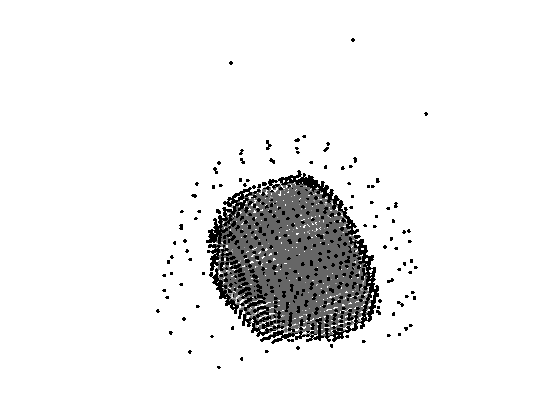Figure: The sensor positions, the source model and the head model nicely align up. Note that the front of the brain is located where the helmet opens up (which it does at the front).

When all these align up well, we have all the geometric ingredients for source analysis. In the next steps, we need to incorporate the actually measured functional data.

#### Exercise: averaging over subjects

What would be the consequence of averaging over subject specific grids?

## Localization of sources of oscillatory gamma-band activity

The aim is to identify the sources of oscillatory activity in the gamma band. In the section time-frequency analysis we have identified the frequency band around 40 Hz to 70 Hz with a center frequency of about 55 Hz. We seek to compare the activation during the post-stimulus interval to the activation during the pre-stimulus interval. We will use ft_redefinetrial to extract relevant data. Remember that the length of each data piece has to be the length of a fixed integer number of oscillatory cycles. Here we select a time window of 0.8s, which allows for an integer amount of cycles: 0.8 s*55 Hz = 44 cycles. Thus, the pre-stimulus time-window ranges from -0.8 s to 0.0 s and the post-stimulus interval between 0.3 s to 1.1 s (see Figure 1).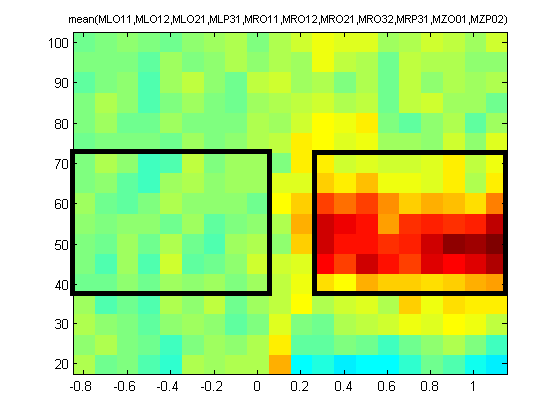Figure: The time-frequency presentation used to determine the time- and frequency-windows prior to beamforming. The squares indicate the selected time-frequency tiles for the pre- and post-response!.

#### Exercise: data length

Why does the length of each data piece has to have the length of a fixed number of oscillatory cycles?

### Time windows of interest

We already combined data from the left-hand response condition and the right-hand response condition (see above). Now we need to make sure that all trials to be analyzed have data in the time interval of interest (if not, we remove those trials).

``````cfg           = [];
cfg.toilim    = [-0.8 1.1];
cfg.minlength = 'maxperlen'; % this ensures all resulting trials are equal length
data          = ft_redefinetrial(cfg, data_combined);
``````

Now, we ‘cut’ out the pre- and post-stimulus time windows:

``````cfg        = [];
cfg.toilim = [-0.8 0];
data_bsl   = ft_redefinetrial(cfg, data);

cfg.toilim = [0.3 1.1];
data_exp   = ft_redefinetrial(cfg, data);
``````

As mentioned in the Background, it is ideal to contrast the activity of interest against some control.

1. Suitable control windows are, for example:
• Activity contrasted with baseline (example shown here using data_bsl)
• Activity of condition 1 contrasted with condition 2 (using e.g., data_left and data_right)
2. However, if no other suitable data condition or baseline time-window exists, then options are:
• Activity contrasted with estimated noise

The latter two cases are covered in another tutorial that we will not deal with today.

The null hypothesis for both options in (1) is that the data (thus also the noise-level) in these conditions are the same, and thus the best spatial filter is the one computed using both these conditions together (also known as ‘common filters’). This common filter is then applied separately to each condition.

In order to not run every function twice, we can combine the two data structure for now. Note that we have to keep track of the condition of each trial, which we will code in the `.trialinfo` field (you could code it in any variable, but for consistency with the FieldTrip coding style and structure, we strongly advise you to use the `.trialinfo` field).

``````cfg      = [];
data_cmb = ft_appenddata(cfg, data_bsl, data_exp);

% give a number to each trial: 0 = baseline, 1 = experimental condition
data_cmb.trialinfo = [zeros(length(data_bsl.trial), 1); ones(length(data_exp.trial), 1)];
``````

### Calculating the cross spectral density matrix

The beamformer technique is based on an adaptive spatial filter. The DICS spatial filter is derived from the frequency counterpart of the covariance matrix: the cross-spectral density matrix. This matrix contains the cross-spectral densities for all sensor combinations and is computed from the Fourier transformed data of the single trials. It is given as output when `cfg.output = 'powandcsd'`, but we can also use `cfg.output = 'fourier'` for that (the CSD will then be inferred from the Fourier coeffcients). Since the frequency band we identified on sensor level ranged from about 40 Hz to 70 Hz, we select the frequency of interest as 55 Hz and a smoothing window of +/-15 Hz:

``````cfg            = [];
cfg.method     = 'mtmfft';
cfg.output     = 'fourier';
cfg.keeptrials = 'yes';
cfg.tapsmofrq  = 15;
cfg.foi        = 55;
freq_cmb       = ft_freqanalysis(cfg, data_cmb);
``````

Now, we can separate the two conditions again:

``````cfg                = [];
cfg.trials         = freq_cmb.trialinfo == 0;
freq_bsl           = ft_selectdata(cfg, freq_cmb);

cfg.trials         = freq_cmb.trialinfo == 1;
freq_exp           = ft_selectdata(cfg, freq_cmb);
``````

Note that we will need all three data structures for beamforming later on, so keep them.

Before computing the leadfields, we need to load again our source- and headmodels if they are not in memory anymore:

``````load hdm
``````

Since we already verified that sensors, head- and sourcemodel align up, we can continue to computing the leadfield matrices by incorporating our just computed frequency data. Note that this step only uses the gradiometer definition and the identity of the channels present in the data, but not the actual data itself. Therefore the resulting leadfield can be used with any subsequent source analysis step that uses data from the same recording session.

``````cfg             = [];
cfg.grid        = sourcemodel;
cfg.channel     = {'MEG'};
``````

If you are not contrasting the activity of interest against another condition or baseline time-window, then you may choose to normalize the lead field in this step (cfg.normalize=’yes’), which will help control against the power bias towards the center of the head.

### Source analysis and contrasting conditions

Using the cross-spectral density and the lead field matrices a spatial filter is calculated for each grid point. By applying the filter to the Fourier transformed data we can then estimate the power for the pre- and post-stimulus activity. This results in a power estimate for each grid point. Since we want to use a common filter, we first need to input data from all conditions:

``````cfg                   = [];
cfg.frequency         = freq_cmb.freq;
cfg.method            = 'dics';
cfg.keeptrials        = 'yes';
cfg.channel           = 'MEG';
cfg.sourcemodel       = sourcemodel_lf;
cfg.keeptrials        = 'yes';
cfg.dics.lambda       = '5%';
cfg.dics.keepfilter   = 'yes';
cfg.dics.fixedori     = 'yes';
cfg.dics.realfilter   = 'yes';
source                = ft_sourceanalysis(cfg, freq_cmb);
``````

The purpose of cfg.fixedori is that we only keep the largest of the three dipole directions per spatial filter and cfg.realfilter specifies that we do not allow our filter to have an imaginary part. The purpose of lambda is discussed in Exercise 6. By using cfg.keepfilter = ‘yes’, we let ft_sourceanalysis return the filter matrix in the source structure.

#### Exercise: complex numbers

What would keeping a complex-valued filter imply for the mapping from sources to sensors?

### Plotting sources of oscillatory gamma-band activity

When plotting the source-level power now, you would realize that the power is strongest in the center of the brain. As already mentioned, there are several ways of circumventing the noise bias towards the center of the head. The most intuitive and powerful approach is to contrast two conditions, which may also be a baseline and an experimental condition as we are dealing with here.

Remember that we intended to contrast the baseline with the experiment time period. Therefore, we need to estimate activity on the source level for the baseline data and for the experiment data using the filter obtained from beaming data from both conditions (‘common filter’

``````% beam pre- and poststim by using the common filter
cfg.sourcemodel.filter  = source.avg.filter;
cfg.sourcemodel.label   = source.avg.label;
source_bsl       = ft_sourceanalysis(cfg, freq_bsl);
source_exp       = ft_sourceanalysis(cfg, freq_exp);
``````

Now we can finally compute the difference between the two conditions. Here we take the ratio between the two conditions centered around 0, so that we obtain the relative difference of the experimental condition from the baseline condition in percent. In this operation we assume that the noise bias is the same for the baseline and experimental stimulus interval and it will thus cancel out when contrasting.

``````cfg = [];
cfg.parameter = 'avg.pow';
cfg.operation = '(x1 ./ x2) - 1';
source_diff = ft_math(cfg, source_exp, source_bsl);
``````

After successfully applying the above steps, we have now obtained an estimate of the difference in the gamma frequency band between the baseline and the experimental time interval at each grid point in the brain volume. The grid of estimated power values can be plotted superimposed on the anatomical MRI. This requires the output of ft_sourceanalysis to match the coordinate system of the MRI on which we want to display the results. Because we based our source model on a regular grid in MNI space, we can simply overwrite the subject-specific grid position information with the corresponding MNI coordinates:

``````source_diff.pos = template.sourcemodel.pos;
source_diff.dim = template.sourcemodel.dim;
``````

The function ft_sourceinterpolate interpolates the source level activity onto an anatomical MRI. We only need to specify what parameter we want to interpolate and to specify the MRI we want to use for interpolation. Here, we again use the template MRI. For reading in the template MRI, you can just use ft_read_mri. That template is distributed with SPM and also is in the fieldtrip/external/spm8 directory:

``````templatedir = fullfile(ftdir, 'external', 'spm8', 'templates');
template_mri.coordsys = 'mni'; % we know it's in MNI space

cfg              = [];
cfg.parameter    = 'pow';
cfg.interpmethod = 'nearest';
source_diff_int  = ft_sourceinterpolate(cfg, source_diff, template_mri);
``````

Now, we can plot the interpolated data:

``````cfg               = [];
cfg.method        = 'slice';
cfg.funparameter  = 'pow';
cfg.funcolorlim   = [0.0 1.2];
cfg.opacitylim    = [0.0 1.2];
cfg.opacitymap    = 'rampup';
ft_sourceplot(cfg, source_diff_int);
``````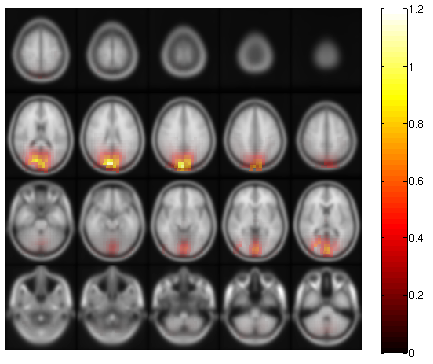Figure: The power estimates of the activity induced by the visual stimulus around 55 Hz.

Congratulations, you successfully beamed visual gamma!

#### Exercise: interpolation options

You may have noticed that the MNI template brain is a bit blurry. Can you think of why this is? You could also interpolate your results onto the individual subject’s anatomical MRI. Try this out.

#### Exercise: plotting options

The ‘slice’ method is not the only plotting method implemented. Use the ‘help’ of ft_sourceplot to find what other methods there are and plot the source level results. What are the benefits and drawbacks of the various plotting routines?

Use these settings for ‘surface’ plotting

``````cfg.projmethod     = 'nearest';
cfg.surffile       = 'surface_white_both.mat';
cfg.surfdownsample = 10;
``````

#### Exercise: determining anatomical labels

If you were to name the anatomical label of the source of this visual gamma, what you say? What plotting method is most appropriate for this?

With the use of `cfg.atlas` (only available with `cfg.method = 'ortho'`) you can specify a lookup atlas, which ft_sourceplot will use to return appropriate anatomical labels. One for the MNI template is distributed with FieldTrip and can be found in ‘fieldtrip/template/atlas/aal/ROI_MNI_V4.nii’.

#### Exercise: regularization

The regularization parameter was lambda = ‘5%’. Change it to 0 or to ‘50%’ and plot the power estimate with respect to baseline. How does the regularization parameter affect the properties of the spatial filter?

## Localization of cortical sources that are coherent with the EMG

As explained for this data in the sensor analysis tutorial, the subjects had to extend both their wrists after cue onset, producing a strong beta-band coherence between some MEG and EMG sensors. In the sensor analysis tutorial, we already localized the sensor location of this coherence in the above linked tutorial. In order to localise the neuronal sources which are coherent with the EMG, we can apply beamformers to the data. In fact, the DICS (“Dynamic Imaging of Coherent Sources”) algorithm was specifically formulated in order to localize sources coherent with some (external) signal. In this example, we are going to use the DICS algorithm to estimate the activity of the neuronal sources and to simultaneously estimate the coherence with the EMG. In order to achieve this, we now need an estimate of the cross-spectral density between all MEG-channel combinations, and between the MEG-channels and the EMG, at a frequency of interest. This requires the preprocessed data, see above, or download the file subjectK.mat from our download server.

### Time window of interest

The experiment we got the data from was conducted to examine whether connectivity between the cortex and the muscle is altered in reaction to a response cue (recall that subjects were cued to respond either with the left or right hand at the beginning of each trial, but that both hands were lifted). We will now look at corticomuscular coherence irrespective of the response cue, and simply pool the two conditions. The cleanest time window in which to estimate this effect is the baseline window, with no visual stimulation present. Therefore, we subselect the baseline data to subject to our coherence analysis:

``````cfg                 = [];
cfg.toilim          = [-1 -0.0025];
cfg.minlength       = 'maxperlen'; % this ensures all resulting trials are equal length
data_stim           = ft_redefinetrial(cfg, data);
``````

### Computing the cross-spectral density matrix

Compute the cross-spectral density matrix for 20 +/- 5 Hz:

``````cfg                 = [];
cfg.output          = 'powandcsd';
cfg.method          = 'mtmfft';
cfg.taper           = 'dpss';
cfg.tapsmofrq       = 5;
cfg.foi             = 20;
cfg.keeptrials      = 'yes';
cfg.channel         = {'MEG' 'EMGlft' 'EMGrgt'};
cfg.channelcmb      = {'MEG' 'MEG'; 'MEG' 'EMGlft'; 'MEG' 'EMGrgt'};
freq_csd            = ft_freqanalysis(cfg, data_stim);
``````

### Source analysis

Once we computed this, we can use ft_sourceanalysis using the following configuration. This step requires the subject’s head- and sourcemodel that we both computed above.

``````% if not yet in memory

cfg                 = [];
cfg.method          = 'dics';
cfg.refchan         = 'EMGlft';
cfg.frequency       = 20;
cfg.sourcemodel     = sourcemodel;
source_coh_lft      = ft_sourceanalysis(cfg, freq_csd);
``````

If you input a sourcemodel on which you have not already computed the leadfield matrices, ft_sourceanalysis will compute the leadfield matrices itself first. This step typically takes longer than the actual inverse computation, so it often is wise to precompute leadfields, as we have done above.

### Plotting cortico-muscular coherent sources

In order to be able to visualise the result with respect to the anatomical MRI, we have to do the exact same step as described above, just this time we have to interpolate the coherence parameter rather than the power parameter:

``````source_coh_lft.pos = template.sourcemodel.pos;
source_coh_lft.dim = template.sourcemodel.dim;

cfg              = [];
cfg.parameter    = 'coh';
cfg.interpmethod = 'nearest';
cfg.coordsys     = 'mni';
source_coh_int   = ft_sourceinterpolate(cfg, source_coh_lft, template_mri);
``````

Again there are various ways to visualise the volumetric interpolated data. The most straightforward way is using ft_sourceplot.

``````cfg               = [];
cfg.method        = 'ortho';
cfg.funparameter  = 'coh';
ft_sourceplot(cfg, source_coh_int);
``````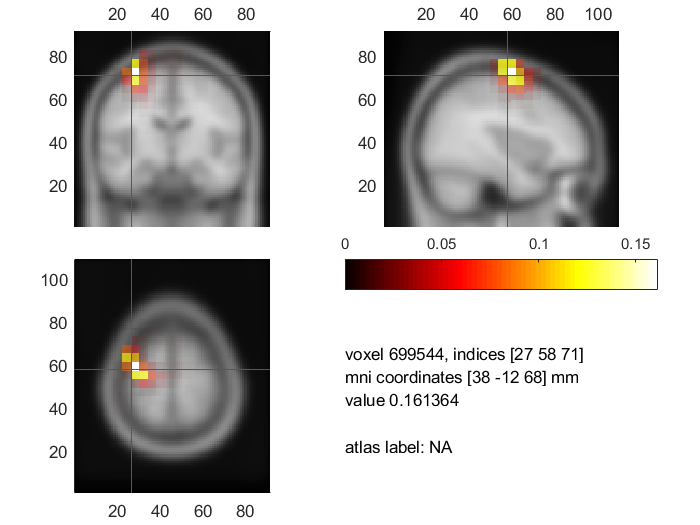Figure: The neuronal source showing maximum coherence with the left EMG at 20 Hz. The plot was created with ft_sourceplot.

Since the data is expressed in MNI coordinates, you can also make a surface rendering of the coherence displayed on the cortical sheet:

``````cfg               = [];
cfg.method        = 'surface';
cfg.funparameter  = 'coh'; % use it to represent color
cfg.maskparameter = 'coh'; % and use it for transparency
ft_sourceplot(cfg, source_coh_int);
``````

#### Note and exercise: flipped axes?

The template MRI included with SPM (and therefore with FieldTrip) is oriented such that the anatomical left is projected to the right side of the screen, and vice versa. This is why the volumetric above are also oriented as such. You could download a version of the MNI brain (see bottom of that page, use NIFTI format, file ‘average305_t1_tal_lin.nii’ in the resulting ZIP) that is oriented differently, and use it instead, if you want to prevent this.

#### Exercise: anatomical labeling

Determine the anatomical location of the coherence peak. How does this result compare to coherence with the right EMG?

#### Exercise: comparison with sensor level analysis

How do all these beamforming result relate to the sensor level analysis?

## Summary

We demonstrated how to apply the DICS beamformer algorithm in the frequency domain. The essence of a source reconstruction model requires to compute a head- and sourcemodel to derive the leadfields. Here, we showed how to compute a head model (single shell) and forward lead field based on an anatomical template in MNI space. Then, we applied the beamformer to retrieve activity on source level. We interpolated the source level result and plotted it against the template anatomy. Subsequently, options for plotting on slices, orthogonal views, or on the surface were shown. In a next step, we discussed how to identify sources of cortico-muscular coherence using the nearly exact pipeline as for ordinary source reconstruction.

Details on head models can be found here or here. Another tutorial on beamforming that covers options without contrasting conditions can be found here. Computing event-related fields with MNE or LCMV might be of interest. More information on common filters can be found here. See here for source statistics. If you want to dive deeper into coherence, take a look here. And in the appendix there is a way described how to compute virtual MEG sensors.